4 분 소요

# INTRO 🙌

저번 시간에는 Transaction Management 에 대하여 알아보았다.

이번 시간에는 Normalization에 대해 알아보자.

# 배경지식 🍔

## DB Design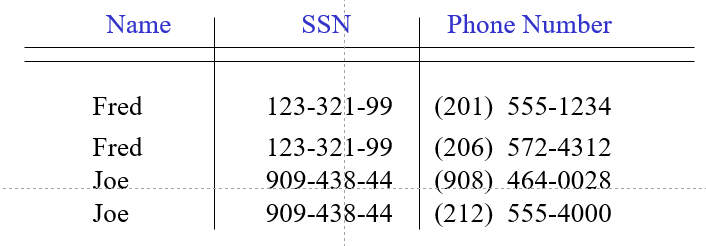• Relation may not be well-designed, thus causing us a lot of problems
• Problems(= Anomalies):
• Redundancy: repetition of data
• update anomalies
• update one item and forget others
• inconsistencies
• deletion anomalies
• delete many items
• delete one item, loose other information
• insertion anomalies
• can’t insert one item without inserting others

## Good Design

[Better Design: Break the relation into two]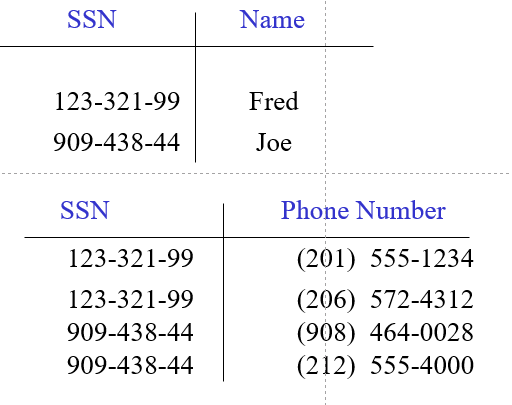• Original db schema R 사용
• Good design R* 얻을 때까지 반복
• R* 이상적인 조건
• R 정보 포함
• Redundancy ↓
• Dependency-preserving; R $$\leftrightarrow$$ constraints C $$\rightarrow$$ C $$\leftrightarrow$$ R*
• Good query performance

그렇다면, 어떻게 좋은 R* 찾아낼 수 있을까?

상기 문제를 해결하고자 고안된 것이 바로 normal forms이다.

# Normal Forms 🍚

• DB gurus:
• Normal forms (Boyce-Codd, 3rd, and 4th normal forms)
• Based on various constraints
• R* = one of these forms $$\rightarrow$$ R* is guaranteed to achieve certain good properties
• e.g., if R* is in Boyce-Codd NF, it is guaranteed to not have certain types of redundancy
• Algorithms to transform R into R* that is in some of these normal forms
• Our job:
• learn these forms
• transform R into R* in one of these forms

Normalization 목적 ★★★★★★★★★

To find a good relational schema by minimizing redundency

# Relation Keys

• Key of a relation R is a set of attributes (primary key)
• functionally determines all attributes of R
• creates a FD A $$\rightarrow$$ B
• none of its subsets determines all attributes of R
• Superkey $$\Leftrightarrow$$ Key
• a set of attributes that contains a key

## Finding the Keys of a Relation

1. Entity set: the key of the relation = the set of attributes, the key of the entity set.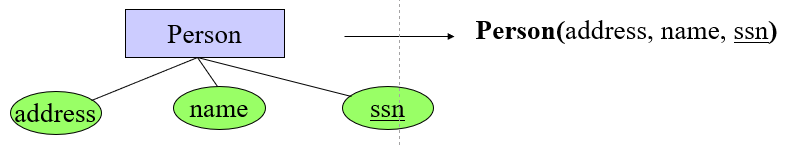1. Many-many relationship: the key of the relation = the set of all attribute keys in the relations corresponding to the entity sets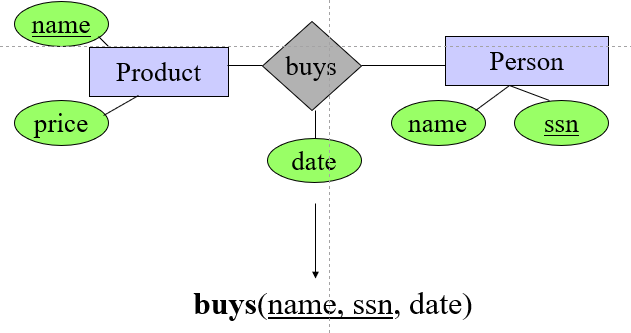1. Many-one, one-many, one-one relationships, Multi-way relationships, Weak entity sets

The set of attributes A is a key $$\rightarrow$$ certain FDs are true.

# Functional Dependencies

$$A \rightarrow B:\ A\ functionally\ determines\ B$$

• A form of constraint (hence, part of the schema)
• Finding them is part of the database design
• Used heavily in schema refinement
• When creating a DB schema, we should list all FDs we believe are valid
• FDs should be valid on ALL DB database instances conforming to our schema

$$A_1,A_2,...,A_n\ \rightarrow\ B_1,B_2,...,B_n$$

• If two tuples agree on the attributes $$A_1,A_2,...,A_n$$, then they must also agree on the attributes $$B_1,B_2,...,B_n$$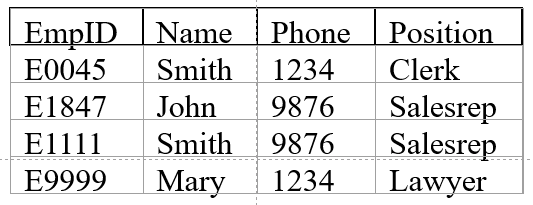$EmpID\ \rightarrow Name,\ Phone,\ Position$ $Position\ \rightarrow\ Phone$ $Phone\ \nrightarrow\ Position$

Phone 1234는 Position에서 ClerkLawyer 두 가지 튜플을 가지기 때문에 dependency가 성립하지 않는 모습이다.

## Functional Dependency 증명

[$$A \rightarrow B$$]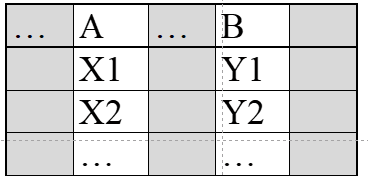1. Erase all other columns
2. Check if $$A\ \Leftrightarrow \ B$$ many-one (called functional in mathematics)

## Reasoning with FDs

### 1) closure of FD sets

Relation Schema R: $$R = {A,B,C,G,H,I}$$

A set S of FDs: $$S = A \rightarrow B, A \rightarrow C, CG \rightarrow H, CG \rightarrow I, B \rightarrow H$$

• Closure of S: S+ = all FDs logically implied by S
• $$A \rightarrow H$$ logically implied
• Compute S+ using Armstrong’s axioms
• For each f in S, apply reflexivity and augment. rules
• Add the new FDs to S+
• For each pair of FDs in S, apply the transitivity rule
• Add the new FD to S+
• Until S+ does not change any further

#### Armstrong’s Axioms

• Reflexivity rule
• $A1A2...An \rightarrow\ a\ subset\ of\ A1A2...An$
• Augmentation rule
• $A1A2...An \rightarrow B1B2...Bm,\ then\ A1A2...An C1C2..Ck \rightarrow B1B2...Bm C1C2...Ck$
• Transitivity rule
• $A1A2...An \rightarrow B1B2...Bm\ and\ B1B2...Bm \rightarrow C1C2...Ck,\ then\ A1A2...An \rightarrow C1C2...Ck$

• Union rule
• $$X \rightarrow Y\ and\ X \rightarrow Z,\ then\ X \rightarrow YZ$$ (X, Y, Z are sets of attributes)
• Decomposition rule
• $X \rightarrow YZ,\ then\ X \rightarrow Y\ and\ X \rightarrow Z$
• Pseudo-transitivity rule
• $X \rightarrow Y\ and\ YZ \rightarrow U,\ then\ XZ \rightarrow U$

### 2) closure of attribute sets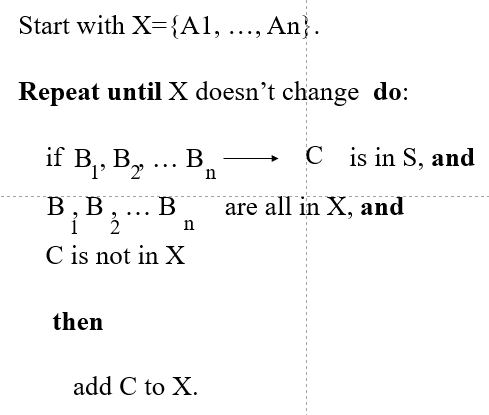The closure of {A1, …, An}, denoted {A1, …, An}+, is the set of all such attributes B

• 1) Functionally determined by A1, …, An
• 2) Satisfies S, a set of FDs S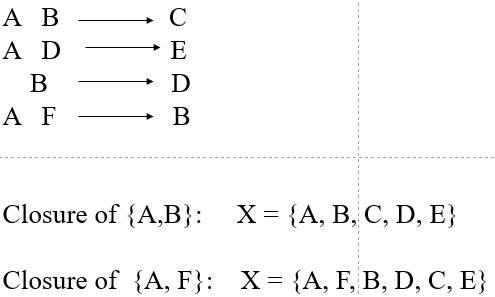# Desirable Properties of Schema Refinement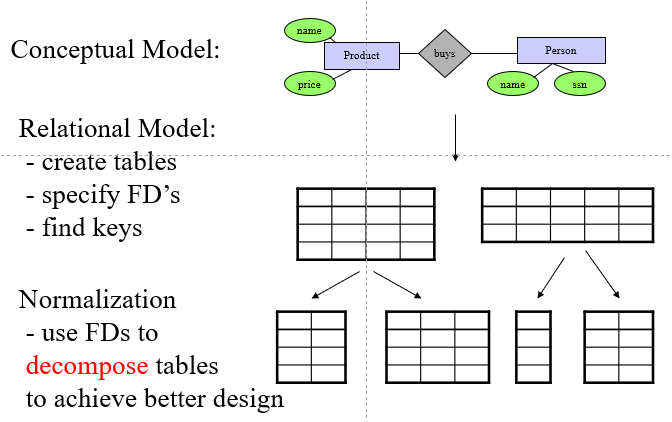1. minimize redundancy
2. avoid info loss (lossless decomposition)
3. preserve dependency
4. ensure good query performance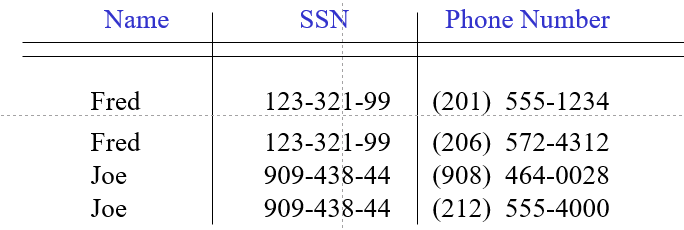## Relation Decomposition

### Desirable Property #1: Minimize redundancy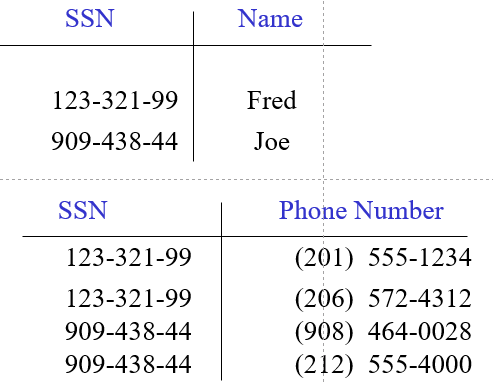분해된 테이블들을 다시 기존 테이블로 변환할 때, 하기 문제점이 발생한다.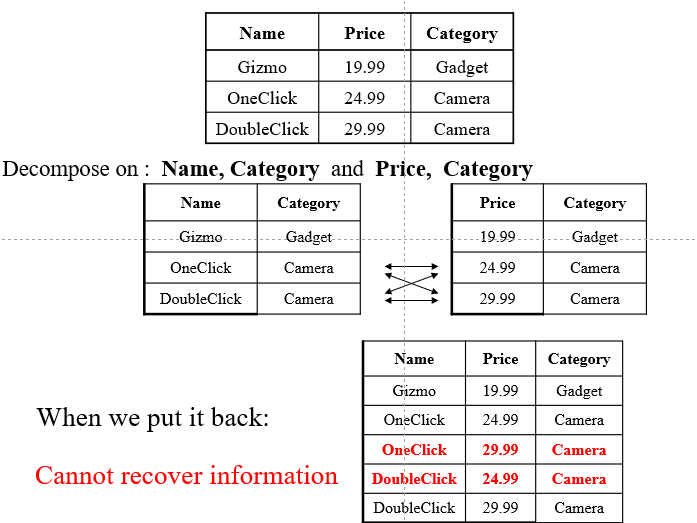# Boyce Codd Normal Form (BCNF)

[BCNF]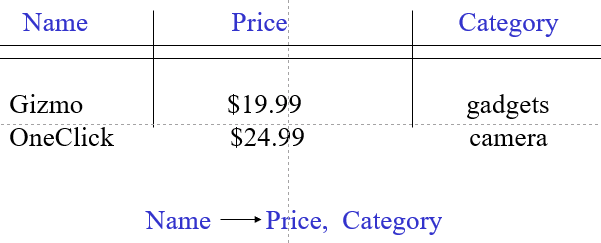A simple condition for removing anomalies from relations:

        Whenever there is a nontrivial FD {A1,A2,...,An --> B}
for  R , it is the case that {A1,A2,...,An --> B}
is a super-key for R.


Super Key

Whenever a set of attributes of R is determining another attribute, it is a super-key, and thus should determine all attributes of R. (A key is also a super-key)

## BCNF Decomposition

• BCNF removes certain types of redundancy
• For examples of redundancy that it cannot remove, see “multivalued redundancy”
• BCNF avoids info loss
1. Find a dependency that violates the BCNF condition:
$A_1, A_2, … A_n \rightarrow B_1, B_2, ..., B_m$
1. Decompose: Continue until there are no BCNF violations left.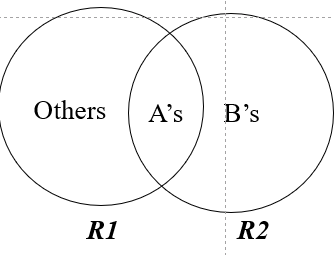Any 2-attribute relation is in BCNF.

## 예시 1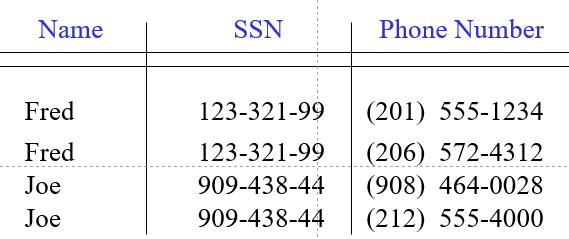What are the FDs?

• $$SSN \rightarrow Name$$.

Does this FD satisfy the BCNF condition?

• No, $$SSN \nrightarrow Phone\ Number$$.

Is the relation in BCNF?

• No

### Decompose it into BCNF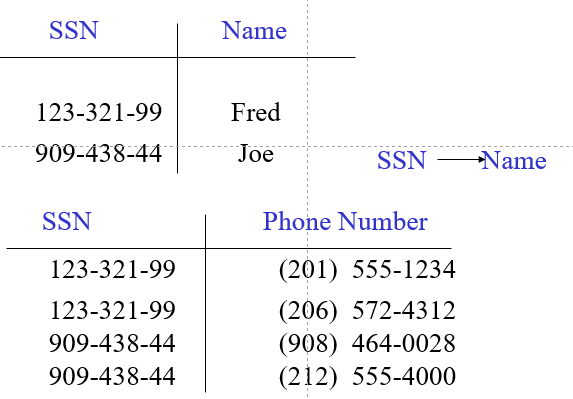• 주어진 FD에서 $$super\ key \rightarrow 나머지$$.
• $$SSN \rightarrow Phone\ Number$$ 추가.

## 예시 2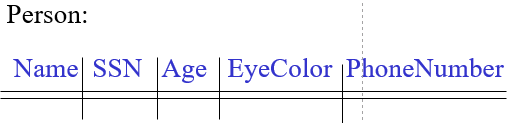FD: $$SSN \rightarrow Name, Age, Eye\ Color$$. BCNF: $$Person1(SSN, Name, Age, EyeColor), Person2(SSN, PhoneNumber)$$.

## 예시 3FD:

• $$SSN \rightarrow Name, Age, Eye\ Color$$.
• $$Age \rightarrow Draft-worthy$$.

BCNF: $$Person1a(SSN,Name,Age,EyeColor),\ Person1b(Age, Draft-worthy),\ Person2(SSN, PhoneNumber)$$

• STEP 1: $$SSN \rightarrow Name, Age, EyeColor, Draft-worthy$$.
• STEP 2: $$SSN \rightarrow Name, Age, EyeColor$$.
• STEP 2: $$Age \rightarrow Draft-worthy$$.
• STEP 1: $$SSN \rightarrow PhoneNumber$$.

더 많은 예제

# Reference

Database Management Systems by Raghu Ramakrishnan and Johannes Gehrke

태그:

카테고리:

업데이트: